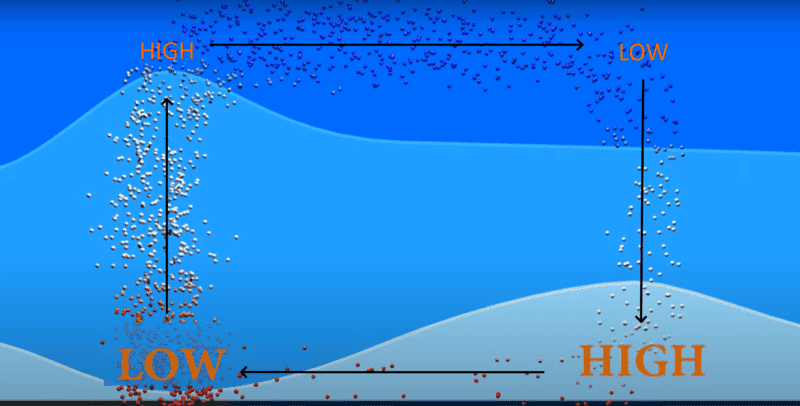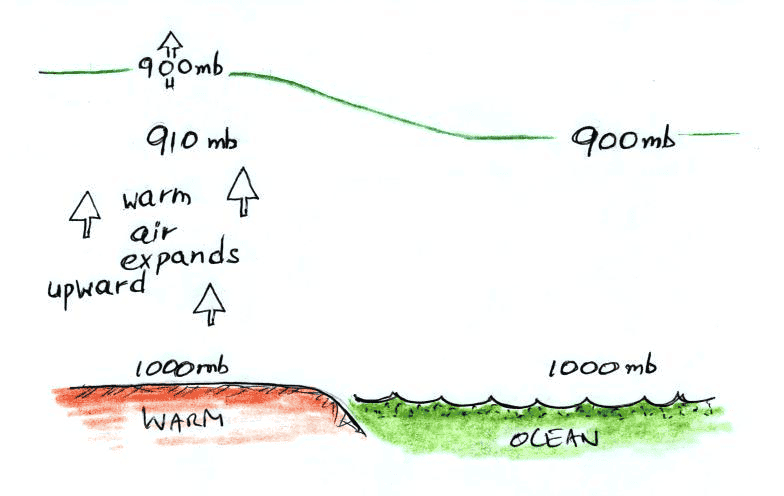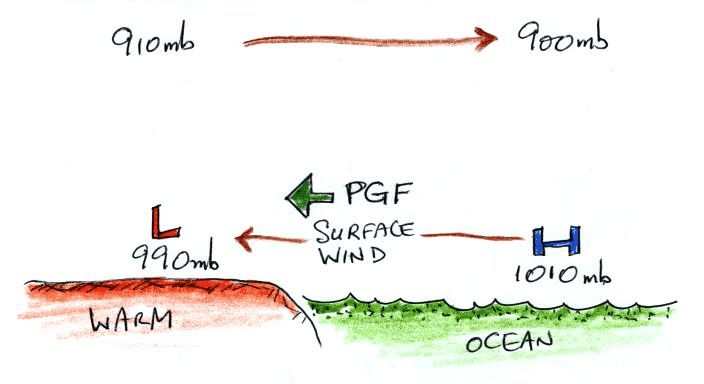# Why does hot air have low pressure? (wind formation)

• I
• jaumzaum

#### jaumzaum

I was studying wind formation from the continent to the sea during day (direct circulation) and the explanation I found in almost all the textbooks was that:
1. In the continent during day the ground becomes hotter
2. The particles in the air increases their temperatures due to induction
3. When the air increases its temperature, the particles become far apart from each other, and the density reduces
4. Due to buoyancy, this air rises
5. As the air rises, for some reason, this creates a low pressure site at the ground and a high pressure site at the sky
6. The air now flows from the sky of the continent (high pressure) to the sky of the ocean (low pressure)
7. To complete the cycle the air drops (as it cools down) to the surface of the ocean creating a high pressure point at sea surface
8. Then it comes back to the continentI can understand all the 8 statements, except the fifth. Why is the continent ground low pressure?

I mean, if we use PV=nRT or better PM = dRT (where M is molar mass and d is density), I know that when T increases d will decrease. But consider T doubles. If d drops less than 50% than P will increase. If d drops 50% P will remain constant. If d drops more than 50% P will decrease. So why the density at the ground drops always more than the increase in temperature, this is not obvious to me. Less density air will have lower pressure, but hotter air will have higher pressure, so the question is, why the first event wins?

Thank you!

Basically, I imagine the sparse hot air is having troubles, both pulling in denser air at the bottom and pushing denser air out at the top.

Meanwhile re: #2 - "conduction" and "radiation", not "induction".

Hotter air would have higher pressure if it were constrained to always occupy the same volume. (For example if it were within a rigid container). That's because the temperature is a measure of the average kinetic energy of the molecules. They'd be moving faster on average, and the number of them would be unchanged, so they'd impart more force to the walls of their container.

But in this case, we have no container. We have a parcel of air in the atmosphere that is experiencing localized heating due to conduction (not induction as you write in step 2) of heat from the hot ground beneath it. For this parcel of air, V is not constant, it's completely free to go up, as a result of the increase in T. So what determines exactly what happens to the thermodynamic state of the parcel of air? I suspect the answer (or a good approximation) is adiabatic expansion, where the air parcel (being a poor conductor) does not have time for any heat to be transferred between it and its surroundings as it expands. For adiabatic expansion P*V^gamma = constant, where gamma is some constant that has do with the properties of the gas molecules. You can derive this result from the ideal gas law, along with the assumption of zero heat transfer. Anyway, this result means that if V goes up, P has to go down. The conceptual explanation for this is that in order to expand, the parcel of air has to do work on its surroundings, and consequently loses internal energy.

•malawi_glenn, DaveE and Lnewqban
I can understand all the 8 statements, except the fifth. Why is the continent ground low pressure?
Why is air drawn up chimney heated from below?? Remember at this scale it takes time (hours) for the winds (at maybe 1m/s) to move air. Same thing here: throw in Coriolis Forces and water and you've got weather!

Hotter air would have higher pressure if it were constrained to always occupy the same volume. (For example if it were within a rigid container). That's because the temperature is a measure of the average kinetic energy of the molecules. They'd be moving faster on average, and the number of them would be unchanged, so they'd impart more force to the walls of their container.

But in this case, we have no container. We have a parcel of air in the atmosphere that is experiencing localized heating due to conduction (not induction as you write in step 2) of heat from the hot ground beneath it. For this parcel of air, V is not constant, it's completely free to go up, as a result of the increase in T. So what determines exactly what happens to the thermodynamic state of the parcel of air? I suspect the answer (or a good approximation) is adiabatic expansion, where the air parcel (being a poor conductor) does not have time for any heat to be transferred between it and its surroundings as it expands. For adiabatic expansion P*V^gamma = constant, where gamma is some constant that has do with the properties of the gas molecules. You can derive this result from the ideal gas law, along with the assumption of zero heat transfer. Anyway, this result means that if V goes up, P has to go down. The conceptual explanation for this is that in order to expand, the parcel of air has to do work on its surroundings, and consequently loses internal energy.

Thanks @LastScattered1090!

I really meant "conduction", sorry about the misspelling (my native language is not english).
I thought about the process being a adiabatic, but this also does not make sense.

Think with me: We can drescribe an adiabatic process by five analogous equations:

##1. PV^{\gamma} = C##
##2. TV^{\gamma-1} = C##
##3. T^{\gamma}/P^{\gamma-1} = C##
##4. P/\rho^{\gamma} = C##
##5. T/\rho^{\gamma-1} = C##

Where ##\rho## is the density. If the volume increases (i.e. density decreases) indeed pressure will decrease (eq. 1 and 4), but this happens when we decrease the temperature (and that is the opposite of what's happening).

In an adiabatic heating, volume drops, density increases and pressure increases.

Why is air drawn up chimney heated from below?? Remember at this scale it takes time (hours) for the winds (at maybe 1m/s) to move air. Same thing here: throw in Coriolis Forces and water and you've got weather!

That's exactly my question! kkkk
It would make sense for me if initially the temperature increases, and so pressure increases. Air will begin to rise because of the pressure gradient (higher below). This will make the pressure drops after some time, because there will be less air in the ground. But what I don't understand is why it drops below the initial pressure?

•Delta2
I think ultimately one needs equations of fluid dynamics, that relate temperature, pressure and density and velocity of air at every point in space and time to describe this system. The full explanation using fluid dynamics won't be that simple but I think you got the main point that the density of air becomes much smaller so that the pressure becomes lower despite temperature being higher.
The why of "density of air becomes much lower at that spot" is something that can be explained fully only by using fluid dynamics. At least that's what I think.

Last edited:
5. As the air rises, for some reason, this creates a low pressure site at the ground and a high pressure site at the sky

I can understand all the 8 statements, except the fifth. Why is the continent ground low pressure?
This may help:
https://en.wikipedia.org/wiki/Stack_effect

You can imagine "the sky" or higher layers of the atmosphere as the top end of the stack.

•Delta2
What gets me is a few hundred thousand feet of air only weighs 14.7psi.

Actually its more like 20,000 ft of STP air. What amazes me is the thinness of the substantive layer.

Yeah, I suppose a ton per sq ft column sounds a bit less fantastical. Given the Internet says air weighs .0807 lbs/cu.ft, so that's more like 24-25k ft of STP.

Last edited:
Now that I think about the figure a bit more carefully, I think the High and Lows labels are relating only horizontally and not vertically, otherwise we would have flow from low pressure spots to high pressure spots.

So we just have to argue why the air at the ground is of lower pressure with regards to the air on the surface of the ocean.

•Lnewqban
•Delta2
Now that I think about the figure a bit more carefully, I think the High and Lows labels are relating only horizontally and not vertically, otherwise we would have flow from low pressure spots to high pressure spots.

So we just have to argue why the air at the ground is of lower pressure with regards to the air on the surface of the ocean.

https://en.wikipedia.org/wiki/Prevailing_winds

https://en.wikipedia.org/wiki/Sea_breeze

https://legacy.climate.ncsu.edu/edu/Breezes

•Delta2
Maybe @boneh3ad or @Chestermiller can provide insight here.
To get a feel for how buoyancy-driven natural convection flows like this work, see Section 10.9 of Transport Phenomena by Bird, et al. Here they consider a viscous fluid between two infinite vertical plates, one at a high temperature and the other at a lower temperature. The temperature distribution between the plates sets up a natural convection circulation in which the flow near the hotter plate is upward and the flow near the colder plate is downward. The flow turns direction at the two infinite ends of the plates.

•vanhees71, Lord Jestocost and Delta2
After reading the book @Chestermiller suggested and other books I think I got a qualitative answer:
As the movement is extremely slow we can say that the pressure in any spot is very close to the hydrostatic pressure in that spot (weight of the air column above).

First, continent ground is heated. This make air become less dense and dilate (upwards and sidewards).
The sidewards dilation contributes to the decrease in the pressure in the continent ground, as it decrease the weight of the air column).
The upwards dilation contributes to the increase in the pressure in the continent sky (as the baroclines will be shifted upwards).
Air then moves to the sky of the continent to the sky of the ocean.
As there are less air in the continent this contributes to a greater decrease of the continent ground pressure.
Some air will be stacked in the ocean now, more weight means more pressure in ocean's surface.•hutchphd, anorlunda, Lnewqban and 1 other person